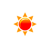# Kilowatt-hour to Joules Converter (kWh to J)

 Example: 1 kilowatt hour = 3 600 000 joules kWh: kWh Joules: J
You may also interested in: Joules to Kilowatt-hour Converter

The online kWh to Joules Converter is used to convert the energy from kWh to Joules.

#### The kWh to Joules Conversion Formula

You can use the following formula to convert from miles to km :

X(J) = y(kWh) × 3600000

Example: How to convert 1 kilowatt-hour to joules ?

X(J) = 1(kWh) × 3600000

#### Kilowatt-hour to joules conversion table


Kilowatt-hour (kWh) Joules (J)
1 kWh3600000 J
2 kWh7200000 J
3 kWh10800000 J
4 kWh14400000 J
5 kWh18000000 J
6 kWh21600000 J
7 kWh25200000 J
8 kWh28800000 J
9 kWh32400000 J
10 kWh36000000 J
20 kWh72000000 J
30 kWh108000000 J
40 kWh144000000 J
50 kWh180000000 J
60 kWh216000000 J
70 kWh252000000 J
80 kWh288000000 J
90 kWh324000000 J
100 kWh360000000 J
500 kWh1800000000 J
1000 kWh3600000000 J

To know how to convert kilowatt hours to Joule, please use our kilowatt hours to Joule Converter for free.

#### References

More references for kilowatt hours and Joule

#### Local Weather Status

Ashburn, US
13th November, 2019 Wednesday
Clear Sky-5 - -1.67
Humidity: 63 %
Wind: 6.2 km/h